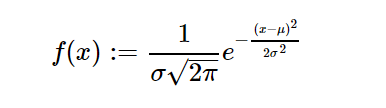Related Articles

# sympy.stats.Normal() in python

• Last Updated : 05 Jun, 2020

With the help of `sympy.stats.Normal()` method, we can get the continuous random variable which represents the normal distribution.Syntax : `sympy.stats.Normal(name, mean, std)`
Where, mean and std are real number.
Return : Return the continuous random variable.

Example #1 :
In this example we can see that by using `sympy.stats.Normal()` method, we are able to get the continuous random variable representing normal distribution by using this method.

 `# Import sympy and Normal``from` `sympy.stats ``import` `Normal, density``from` `sympy ``import` `Symbol, pprint`` ` `z ``=` `Symbol(``"z"``)``mean ``=` `Symbol(``"mean"``, positive ``=` `True``)``std ``=` `Symbol(``"std"``, positive ``=` `True``)`` ` `# Using sympy.stats.Normal() method``X ``=` `Normal(``"x"``, mean, std)``gfg ``=` `density(X)(z)`` ` `pprint(gfg)`

Output :

2
-(-mean + z)
————–
2
___ 2*std
\/ 2 *e
———————
____
2*\/ pi *std

Example #2 :

 `# Import sympy and Normal``from` `sympy.stats ``import` `Normal, density``from` `sympy ``import` `Symbol, pprint`` ` `z ``=` `2``mean ``=` `1.8``std ``=` `4`` ` `# Using sympy.stats.Normal() method``X ``=` `Normal(``"x"``, mean, std)``gfg ``=` `density(X)(z)`` ` `pprint(gfg)`

Output :

0.124843847615573*\/ 2
———————–
____
\/ pi

Attention geek! Strengthen your foundations with the Python Programming Foundation Course and learn the basics.

To begin with, your interview preparations Enhance your Data Structures concepts with the Python DS Course. And to begin with your Machine Learning Journey, join the Machine Learning – Basic Level Course

My Personal Notes arrow_drop_up